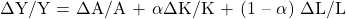Essential Concept 16: Growth Accounting Relations | IFT World
101 Concepts for the Level I Exam

# Essential Concept 16: Growth Accounting RelationsGrowth accounting is used to analyze the performance of economies. The growth accounting equation is as follows:i.e. growth rate of output = rate of technological change + α (growth rate of capital) + (1 – α) (growth rate of labor).
An alternative method of measuring potential GDP is the labor productivity growth accounting equation. Under this approach, the equation for estimating the potential GDP is:
Growth rate in potential GDP = Long-term growth rate of labor force + Long-term growth rate in labor productivity.

The growth accounting equation is used to:

1. Estimate contribution of technological progress
2. Measure sources of growth
3. Measure potential output